# 8   Chart Parsing and Probabilistic Parsing

## 8.1   Introduction

Chapter 7 started with an introduction to constituent structure in English, showing how words in a sentence group together in predictable ways. We showed how to describe this structure using syntactic tree diagrams, and observed that it is sometimes desirable to assign more than one such tree to a given string. In this case, we said that the string was structurally ambiguous; and example was old men and women.

Treebanks are language resources in which the syntactic structure of a corpus of sentences has been annotated, usually by hand. However, we would also like to be able to produce trees algorithmically. A context-free phrase structure grammar (CFG) is a formal model for describing whether a given string can be assigned a particular constituent structure. Given a set of syntactic categories, the CFG uses a set of productions to say how a phrase of some category A can be analyzed into a sequence of smaller parts α1 ... αn. But a grammar is a static description of a set of strings; it does not tell us what sequence of steps we need to take to build a constituent structure for a string. For this, we need to use a parsing algorithm. We presented two such algorithms: Top-Down Recursive Descent (7.5.1) and Bottom-Up Shift-Reduce (7.5.2). As we pointed out, both parsing approaches suffer from important shortcomings. The Recursive Descent parser cannot handle left-recursive productions (e.g., productions such as npnp pp), and blindly expands categories top-down without checking whether they are compatible with the input string. The Shift-Reduce parser is not guaranteed to find a valid parse for the input even if one exists, and builds substructure without checking whether it is globally consistent with the grammar. As we will describe further below, the Recursive Descent parser is also inefficient in its search for parses.

So, parsing builds trees over sentences, according to a phrase structure grammar. Now, all the examples we gave in Chapter 7 only involved toy grammars containing a handful of productions. What happens if we try to scale up this approach to deal with realistic corpora of language? Unfortunately, as the coverage of the grammar increases and the length of the input sentences grows, the number of parse trees grows rapidly. In fact, it grows at an astronomical rate.

Let's explore this issue with the help of a simple example. The word fish is both a noun and a verb. We can make up the sentence fish fish fish, meaning fish like to fish for other fish. (Try this with police if you prefer something more sensible.) Here is a toy grammar for the "fish" sentences.

 ```>>> grammar = nltk.parse_cfg(""" ... S -> NP V NP ... NP -> NP Sbar ... Sbar -> NP V ... NP -> 'fish' ... V -> 'fish' ... """)```

Note

Remember that our program samples assume you begin your interactive session or your program with: import nltk, re, pprint

Now we can try parsing a longer sentence, fish fish fish fish fish, which amongst other things, means 'fish that other fish fish are in the habit of fishing fish themselves'. We use the NLTK chart parser, which is presented later on in this chapter. This sentence has two readings.

 ```>>> tokens = ["fish"] * 5 >>> cp = nltk.ChartParser(grammar, nltk.parse.TD_STRATEGY) >>> for tree in cp.nbest_parse(tokens): ... print tree (S (NP (NP fish) (Sbar (NP fish) (V fish))) (V fish) (NP fish)) (S (NP fish) (V fish) (NP (NP fish) (Sbar (NP fish) (V fish))))```

As the length of this sentence goes up (3, 5, 7, ...) we get the following numbers of parse trees: 1; 2; 5; 14; 42; 132; 429; 1,430; 4,862; 16,796; 58,786; 208,012; ... (These are the Catalan numbers, which we saw in an exercise in Section 5.5). The last of these is for a sentence of length 23, the average length of sentences in the WSJ section of Penn Treebank. For a sentence of length 50 there would be over 1012 parses, and this is only half the length of the Piglet sentence (Section (4)), which young children process effortlessly. No practical NLP system could construct all millions of trees for a sentence and choose the appropriate one in the context. It's clear that humans don't do this either!

Note that the problem is not with our choice of example. [Church & Patil, 1982] point out that the syntactic ambiguity of pp attachment in sentences like (1) also grows in proportion to the Catalan numbers.

 (1) Put the block in the box on the table.

So much for structural ambiguity; what about lexical ambiguity? As soon as we try to construct a broad-coverage grammar, we are forced to make lexical entries highly ambiguous for their part of speech. In a toy grammar, a is only a determiner, dog is only a noun, and runs is only a verb. However, in a broad-coverage grammar, a is also a noun (e.g. part a), dog is also a verb (meaning to follow closely), and runs is also a noun (e.g. ski runs). In fact, all words can be referred to by name: e.g. the verb 'ate' is spelled with three letters; in speech we do not need to supply quotation marks. Furthermore, it is possible to verb most nouns. Thus a parser for a broad-coverage grammar will be overwhelmed with ambiguity. Even complete gibberish will often have a reading, e.g. the a are of I. As [Klavans & Resnik}, 1996] has pointed out, this is not word salad but a grammatical noun phrase, in which are is a noun meaning a hundredth of a hectare (or 100 sq m), and a and I are nouns designating coordinates, as shown in Figure 8.1.Figure 8.1: The a are of I

Even though this phrase is unlikely, it is still grammatical and a a broad-coverage parser should be able to construct a parse tree for it. Similarly, sentences that seem to be unambiguous, such as John saw Mary, turn out to have other readings we would not have anticipated (as Abney explains). This ambiguity is unavoidable, and leads to horrendous inefficiency in parsing seemingly innocuous sentences.

Let's look more closely at this issue of efficiency. The top-down recursive-descent parser presented in Chapter 7 can be very inefficient, since it often builds and discards the same sub-structure many times over. We see this in Figure 8.1, where a phrase the block is identified as a noun phrase several times, and where this information is discarded each time we backtrack.

Note

You should try the recursive-descent parser demo if you haven't already: nltk.draw.srparser.demo()

Table 8.1:

Backtracking and Repeated Parsing of Subtrees

 Initial stage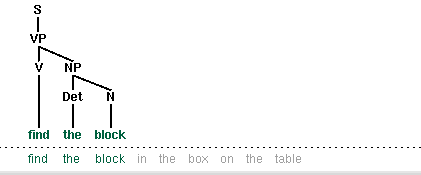Backtracking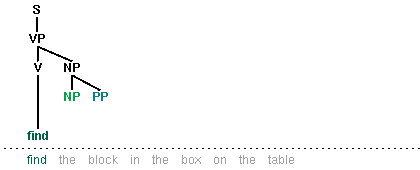Failing to match on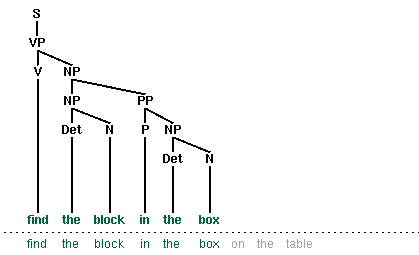Completed parse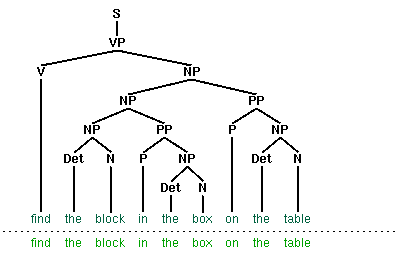In this chapter, we will present two independent methods for dealing with ambiguity. The first is chart parsing, which uses the algorithmic technique of dynamic programming to derive the parses of an ambiguous sentence more efficiently. The second is probabilistic parsing, which allows us to rank the parses of an ambiguous sentence on the basis of evidence from corpora.

## 8.2   Chart Parsing

In the introduction to this chapter, we pointed out that the simple parsers discussed in Chapter 7 suffered from limitations in both completeness and efficiency. In order to remedy these, we will apply the algorithm design technique of dynamic programming to the parsing problem. As we saw in Section 5.5.3, dynamic programming stores intermediate results and re-uses them when appropriate, achieving significant efficiency gains. This technique can be applied to syntactic parsing, allowing us to store partial solutions to the parsing task and then look them up as necessary in order to efficiently arrive at a complete solution. This approach to parsing is known as chart parsing, and is the focus of this section.

### 8.2.1   Well-Formed Substring Tables

Let's start off by defining a simple grammar.

 ```>>> grammar = nltk.parse_cfg(""" ... S -> NP VP ... PP -> P NP ... NP -> Det N | NP PP ... VP -> V NP | VP PP ... Det -> 'the' ... N -> 'kids' | 'box' | 'floor' ... V -> 'opened' ... P -> 'on' ... """)```

As you can see, this grammar allows the vp opened the box on the floor to be analyzed in two ways, depending on where the pp is attached.

(2)

 a.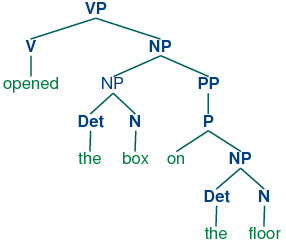b.Figure 8.2: Slice Points in the Input String

This allows us to say that, for instance, the word opened spans (2, 3) in the input. This is reminiscent of the slice notation:

 ```>>> tokens = ["the", "kids", "opened", "the", "box", "on", "the", "floor"] >>> tokens[2:3] ['opened']```

In a WFST, we record the position of the words by filling in cells in a triangular matrix: the vertical axis will denote the start position of a substring, while the horizontal axis will denote the end position (thus opened will appear in the cell with coordinates (2, 3)). To simplify this presentation, we will assume each word has a unique lexical category, and we will store this (not the word) in the matrix. So cell (2, 3) will contain the entry v. More generally, if our input string is a1a2 ... an, and our grammar contains a production of the form Aai, then we add A to the cell (i-1, i).

So, for every word in tokens, we can look up in our grammar what category it belongs to.

 ```>>> grammar.productions(rhs=tokens) [V -> 'opened']```

For our WFST, we create an (n-1) × (n-1) matrix as a list of lists in Python, and initialize it with the lexical categories of each token, in the init_wfst() function in Listing 8.1. We also define a utility function display() to pretty-print the WFST for us. As expected, there is a v in cell (2, 3).

 ```def init_wfst(tokens, grammar): numtokens = len(tokens) wfst = [['.' for i in range(numtokens+1)] for j in range(numtokens+1)] for i in range(numtokens): productions = grammar.productions(rhs=tokens[i]) wfst[i][i+1] = productions.lhs() return wfst def complete_wfst(wfst, tokens, trace=False): index = {} for prod in grammar.productions(): index[prod.rhs()] = prod.lhs() numtokens = len(tokens) for span in range(2, numtokens+1): for start in range(numtokens+1-span): end = start + span for mid in range(start+1, end): nt1, nt2 = wfst[start][mid], wfst[mid][end] if (nt1,nt2) in index: if trace: print "[%s] %3s [%s] %3s [%s] ==> [%s] %3s [%s]" % \ (start, nt1, mid, nt2, end, start, index[(nt1,nt2)], end) wfst[start][end] = index[(nt1,nt2)] return wfst def display(wfst, tokens): print '\nWFST ' + ' '.join([("%-4d" % i) for i in range(1, len(wfst))]) for i in range(len(wfst)-1): print "%d " % i, for j in range(1, len(wfst)): print "%-4s" % wfst[i][j], print```
 ```>>> wfst0 = init_wfst(tokens, grammar) >>> display(wfst0, tokens) WFST 1 2 3 4 5 6 7 8 0 Det . . . . . . . 1 . N . . . . . . 2 . . V . . . . . 3 . . . Det . . . . 4 . . . . N . . . 5 . . . . . P . . 6 . . . . . . Det . 7 . . . . . . . N >>> wfst1 = complete_wfst(wfst0, tokens) >>> display(wfst1, tokens) WFST 1 2 3 4 5 6 7 8 0 Det NP . . S . . S 1 . N . . . . . . 2 . . V . VP . . VP 3 . . . Det NP . . NP 4 . . . . N . . . 5 . . . . . P . PP 6 . . . . . . Det NP 7 . . . . . . . N```

Listing 8.1 (wfst.py): Acceptor Using Well-Formed Substring Table (based on CYK algorithm)

Returning to our tabular representation, given that we have det in cell (0, 1), and n in cell (1, 2), what should we put into cell (0, 2)? In other words, what syntactic category derives the kids? We have already established that Det derives the and n derives kids, so we need to find a production of the form Adet n, that is, a production whose right hand side matches the categories in the cells we have already found. From the grammar, we know that we can enter np in cell (0,2).

More generally, we can enter A in (i, j) if there is a production AB C, and we find nonterminal B in (i, k) and C in (k, j). Listing 8.1 uses this inference step to complete the WFST.

Note

To help us easily retrieve productions by their right hand sides, we create an index for the grammar. This is an example of a space-time trade-off: we do a reverse lookup on the grammar, instead of having to check through entire list of productions each time we want to look up via the right hand side.

We conclude that there is a parse for the whole input string once we have constructed an s node that covers the whole input, from position 0 to position 8; i.e., we can conclude that s ⇒* a1a2 ... an.

Notice that we have not used any built-in parsing functions here. We've implemented a complete, primitive chart parser from the ground up!

### 8.2.2   Charts

By setting trace to True when calling the function complete_wfst(), we get additional output.

 ```>>> wfst1 = complete_wfst(wfst0, tokens, trace=True)  Det  N  ==>  NP   Det  N  ==>  NP   Det  N  ==>  NP   V  NP  ==>  VP   P  NP  ==>  PP   NP  VP  ==>  S   NP  PP  ==>  NP   V  NP  ==>  VP   VP  PP  ==>  VP   NP  VP  ==>  S ```

For example, this says that since we found Det at wfst and N at wfst, we can add NP to wfst. The same information can be represented in a directed acyclic graph, as shown in Figure 8.2(a). This graph is usually called a chart. Figure 8.2(b) is the corresponding graph representation, where we add a new edge labeled np to cover the input from 0 to 2.

Table 8.2:

A Graph Representation for the WFST

 Initialized WFSTAdding an np Edge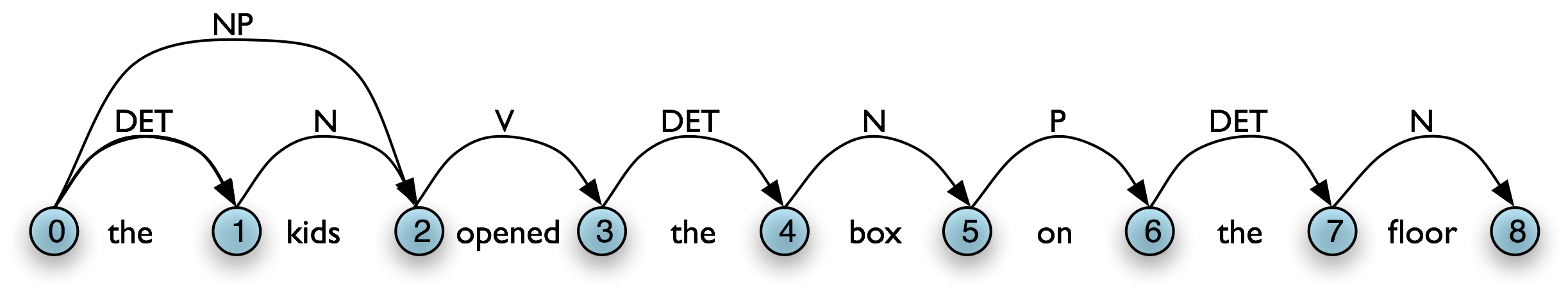(Charts are more general than the WFSTs we have seen, since they can hold multiple hypotheses for a given span.)

A WFST is a data structure that can be used by a variety of parsing algorithms. The particular method for constructing a WFST that we have just seen and has some shortcomings. First, as you can see, the WFST is not itself a parse tree, so the technique is strictly speaking recognizing that a sentence is admitted by a grammar, rather than parsing it. Second, it requires every non-lexical grammar production to be binary (see Section 8.5.1). Although it is possible to convert an arbitrary CFG into this form, we would prefer to use an approach without such a requirement. Third, as a bottom-up approach it is potentially wasteful, being able to propose constituents in locations that would not be licensed by the grammar. Finally, the WFST did not represent the structural ambiguity in the sentence (i.e. the two verb phrase readings). The vp in cell (2,8) was actually entered twice, once for a v np reading, and once for a vp pp reading. In the next section we will address these issues.

### 8.2.3   Exercises

1. ☼ Consider the sequence of words: Buffalo buffalo Buffalo buffalo buffalo buffalo Buffalo buffalo. This is a grammatically correct sentence, as explained at http://en.wikipedia.org/wiki/Buffalo_buffalo_Buffalo_buffalo_buffalo_buffalo_Buffalo_buffalo. Consider the tree diagram presented on this Wikipedia page, and write down a suitable grammar. Normalize case to lowercase, to simulate the problem that a listener has when hearing this sentence. Can you find other parses for this sentence? How does the number of parse trees grow as the sentence gets longer? (More examples of these sentences can be found at http://en.wikipedia.org/wiki/List_of_homophonous_phrases).
2. ◑ Consider the algorithm in Listing 8.1. Can you explain why parsing context-free grammar is proportional to n3?
3. ◑ Modify the functions init_wfst() and complete_wfst() so that the contents of each cell in the WFST is a set of non-terminal symbols rather than a single non-terminal.
4. ★ Modify the functions init_wfst() and complete_wfst() so that when a non-terminal symbol is added to a cell in the WFST, it includes a record of the cells from which it was derived. Implement a function that will convert a WFST in this form to a parse tree.

## 8.3   Active Charts

One important aspect of the tabular approach to parsing can be seen more clearly if we look at the graph representation: given our grammar, there are two different ways to derive a top-level vp for the input, as shown in Table 8.3(a,b). In our graph representation, we simply combine the two sets of edges to yield Table 8.3(c).

Table 8.3:

Combining Multiple Parses in a Single Chart

 vp → v npvp → vp pp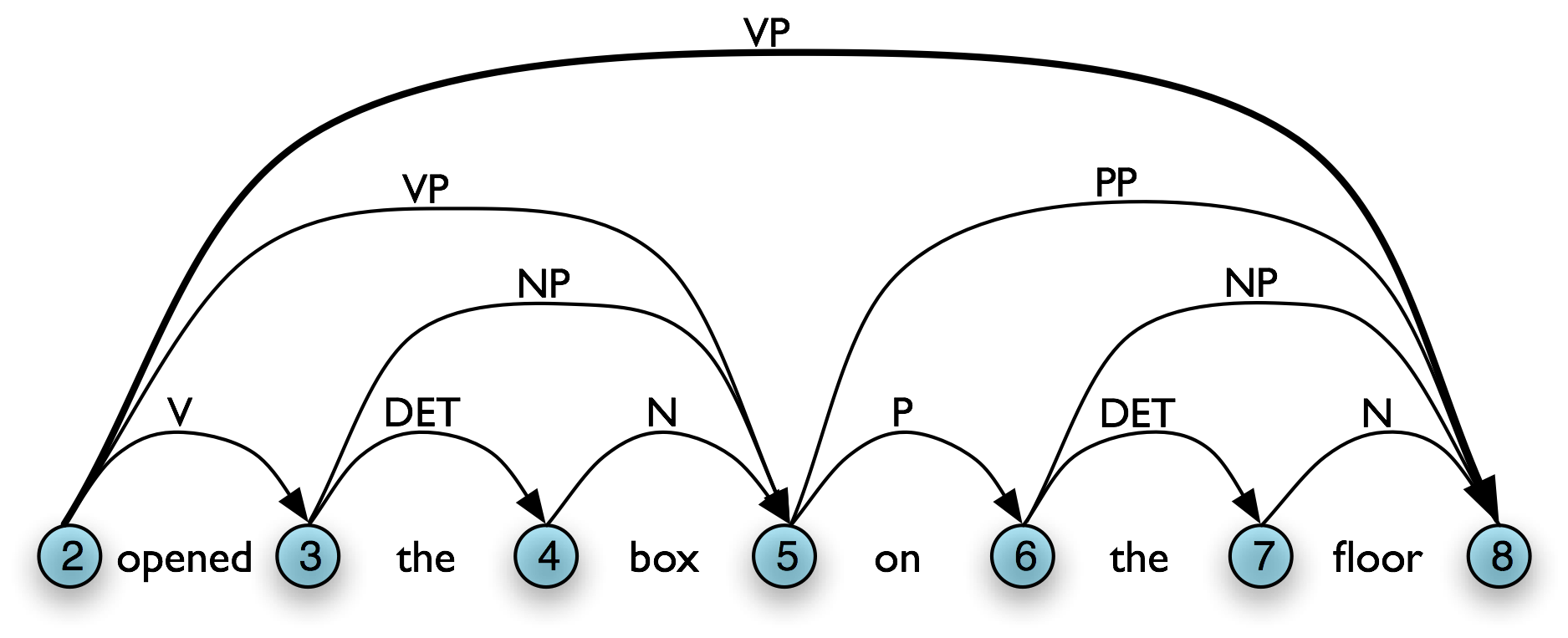Merged ChartHowever, given a WFST we cannot necessarily read off the justification for adding a particular edge. For example, in 8.3(b), [Edge: VP, 2:8] might owe its existence to a production vpv np pp. Unlike phrase structure trees, a WFST does not encode a relation of immediate dominance. In order to make such information available, we can label edges not just with a non-terminal category, but with the whole production that justified the addition of the edge. This is illustrated in Figure 8.3.Figure 8.3: Chart Annotated with Productions

In general, a chart parser hypothesizes constituents (i.e. adds edges) based on the grammar, the tokens, and the constituents already found. Any constituent that is compatible with the current knowledge can be hypothesized; even though many of these hypothetical constituents will never be used in the final result. A WFST just records these hypotheses.

All of the edges that we've seen so far represent complete constituents. However, as we will see, it is helpful to hypothesize incomplete constituents. For example, the work done by a parser in processing the production VPV NP PP can be reused when processing VPV NP. Thus, we will record the hypothesis that "the v constituent likes is the beginning of a vp."

We can record such hypotheses by adding a dot to the edge's right hand side. Material to the left of the dot specifies what the constituent starts with; and material to the right of the dot specifies what still needs to be found in order to complete the constituent. For example, the edge in the Figure 8.4 records the hypothesis that "a vp starts with the v likes, but still needs an np to become complete":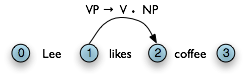Figure 8.4: Chart Containing Incomplete VP Edge

If d = 0, then c1cn is empty and the edge is called a self-loop edge. This is illustrated in Table 8.4(b). If a complete edge spans the entire sentence, and has the grammar's start symbol as its left-hand side, then the edge is called a parse edge, and it encodes one or more parse trees for the sentence. In Table 8.4(c), [snp vp •, (0, 3)] is a parse edge.

Table 8.4:

Chart Terminology

 a. Incomplete Edge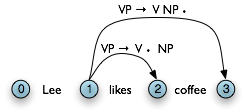b. Self Loop Edgec. Parse Edge### 8.3.1   The Chart Parser

To parse a sentence, a chart parser first creates an empty chart spanning the sentence. It then finds edges that are licensed by its knowledge about the sentence, and adds them to the chart one at a time until one or more parse edges are found. The edges that it adds can be licensed in one of three ways:

1. The input can license an edge. In particular, each word wi in the input licenses the complete edge [wi → •, (i, i+1)].
2. The grammar can license an edge. In particular, each grammar production A → α licenses the self-loop edge [A → • α, (i, i)] for every i, 0 ≤ i < n.
3. The current chart contents can license an edge.

However, it is not wise to add all licensed edges to the chart, since many of them will not be used in any complete parse. For example, even though the edge in the following chart is licensed (by the grammar), it will never be used in a complete parse:Figure 8.5: Chart Containing Redundant Edge

### 8.3.3   Bottom-Up Parsing

As we saw in Chapter 7, bottom-up parsing starts from the input string, and tries to find sequences of words and phrases that correspond to the right hand side of a grammar production. The parser then replaces these with the left-hand side of the production, until the whole sentence is reduced to an S. Bottom-up chart parsing is an extension of this approach in which hypotheses about structure are recorded as edges on a chart. In terms of our earlier terminology, bottom-up chart parsing can be seen as a parsing strategy; in other words, bottom-up is a particular choice of heuristics for adding new edges to a chart.

The general procedure for chart parsing is inductive: we start with a base case, and then show how we can move from a given state of the chart to a new state. Since we are working bottom-up, the base case for our induction will be determined by the words in the input string, so we add new edges for each word. Now, for the induction step, suppose the chart contains an edge labeled with constituent A. Since we are working bottom-up, we want to build constituents that can have an A as a daughter. In other words, we are going to look for productions of the form BA β and use these to label new edges.

Let's look at the procedure a bit more formally. To create a bottom-up chart parser, we add to the Fundamental Rule two new rules: the Bottom-Up Initialization Rule; and the Bottom-Up Predict Rule. The Bottom-Up Initialization Rule says to add all edges licensed by the input.

Table 8.6(a) illustrates this rule using the chart notation, while Table 8.6(b) shows the bottom-up initialization for the input Lee likes coffee.

Table 8.6:

Bottom-Up Initialization Rule

 a. Generic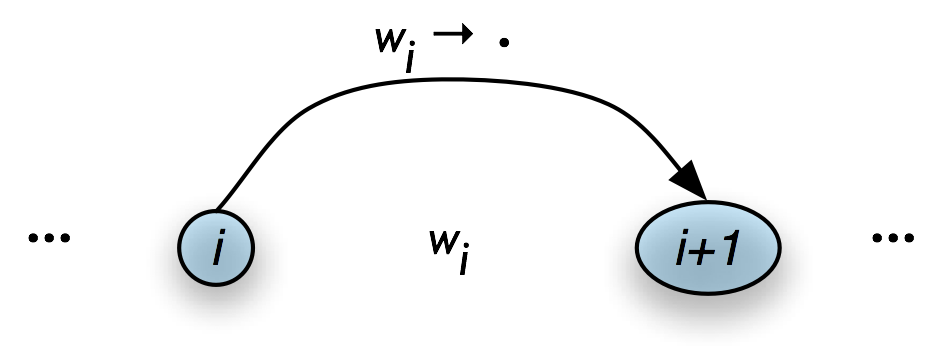b. ExampleNotice that the dot on the right hand side of these productions is telling us that we have complete edges for the lexical items. By including this information, we can give a uniform statement of how the Fundamental Rule operates in Bottom-Up parsing, as we will shortly see.

Next, suppose the chart contains a complete edge e whose left hand category is A. Then the Bottom-Up Predict Rule requires the parser to add a self-loop edge at the left boundary of e for each grammar production whose right hand side begins with category A.

Graphically, if the chart looks as in Figure 8.7(a), then the Bottom-Up Predict Rule tells the parser to augment the chart as shown in Figure 8.7(b).

Table 8.7:

Bottom-Up Prediction Rule

 a. Inputb. Output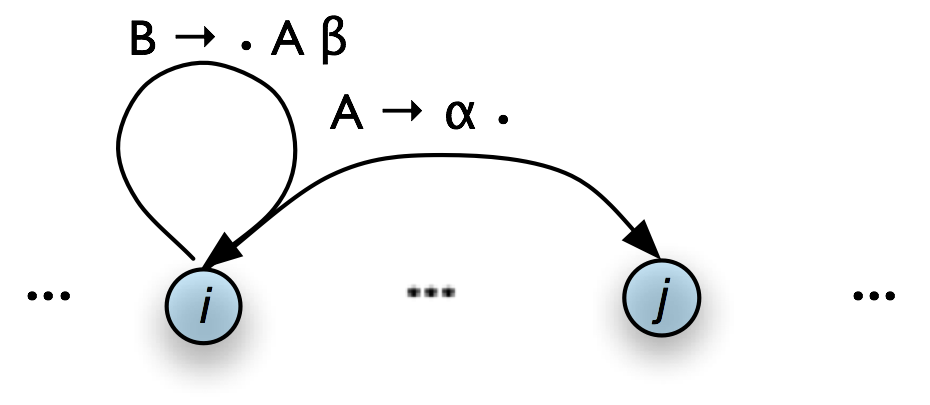To continue our earlier example, let's suppose that our grammar contains the lexical productions shown in (6a). This allows us to add three self-loop edges to the chart, as shown in (6b).

(6)

 a. np → Lee | coffee v → likes

 b.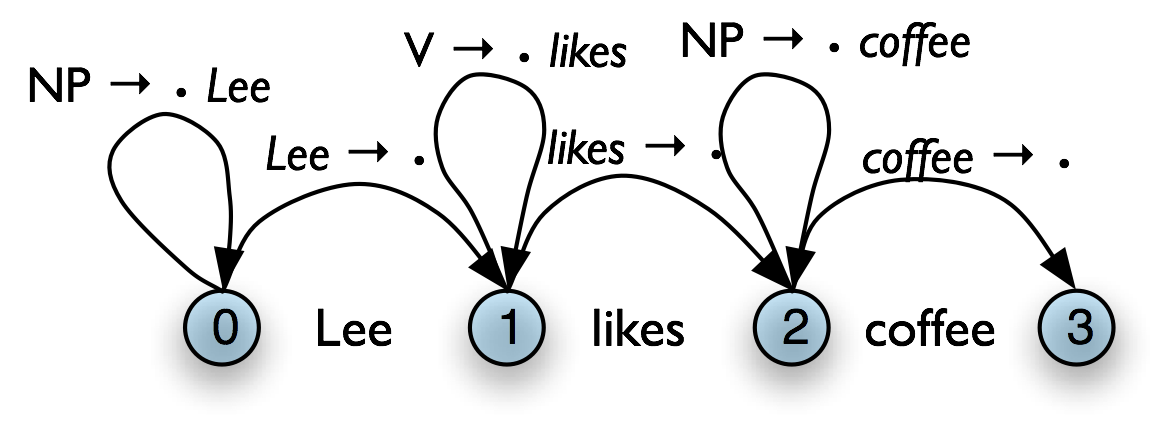Once our chart contains an instance of the pattern shown in Figure 8.7(b), we can use the Fundamental Rule to add an edge where we have "moved the dot" one position to the right, as shown in Figure 8.8 (we have omitted the self-loop edges for simplicity.)

Table 8.8:

Fundamental Rule used in Bottom-Up Parsing

 GenericExampleWe will now be able to add new self-loop edges such as [s → • np vp, (0, 0)] and [vp → • vp np, (1, 1)], and use these to build more complete edges.

Using these three productions, we can parse a sentence as shown in (7).

NLTK provides a useful interactive tool for visualizing the way in which charts are built, nltk.draw.chart.demo(). The tool comes with a pre-defined input string and grammar, but both of these can be readily modified with options inside the Edit menu. Figure 8.6 illustrates a window after the grammar has been updated: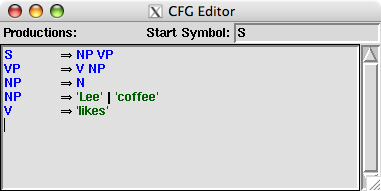Figure 8.6: Modifying the demo() grammar

Note

To get the symbol ⇒ illustrated in Figure 8.6. you just have to type the keyboard characters '->'.

Figure 8.7 illustrates the tool interface. In order to invoke a rule, you simply click one of the green buttons at the bottom of the window. We show the state of the chart on the input Lee likes coffee after three applications of the Bottom-Up Initialization Rule, followed by successive applications of the Bottom-Up Predict Rule and the Fundamental Rule.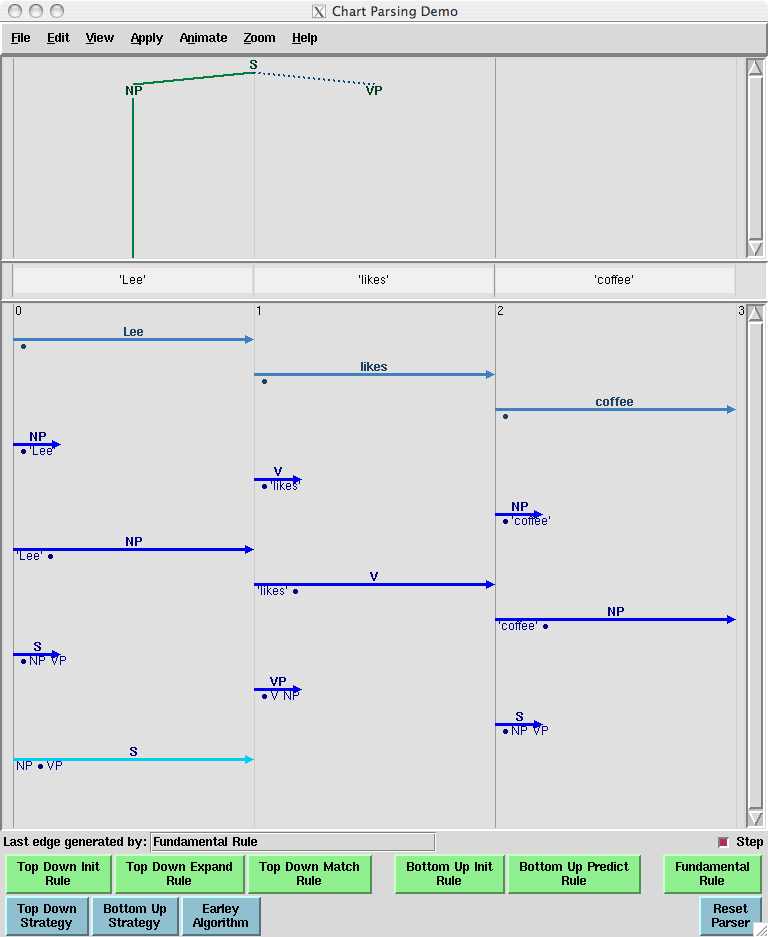Figure 8.7: Incomplete chart for Lee likes coffee

Notice that in the topmost pane of the window, there is a partial tree showing that we have constructed an s with an np subject in the expectation that we will be able to find a vp.

### 8.3.4   Top-Down Parsing

Top-down chart parsing works in a similar way to the recursive descent parser discussed in Chapter 7, in that it starts off with the top-level goal of finding an s. This goal is then broken into the subgoals of trying to find constituents such as np and vp that can be immediately dominated by s. To create a top-down chart parser, we use the Fundamental Rule as before plus three other rules: the Top-Down Initialization Rule, the Top-Down Expand Rule, and the Top-Down Match Rule. The Top-Down Initialization Rule in (8) captures the fact that the root of any parse must be the start symbol s. It is illustrated graphically in Table 8.9.

Table 8.9:

Top-Down Initialization Rule

 GenericExample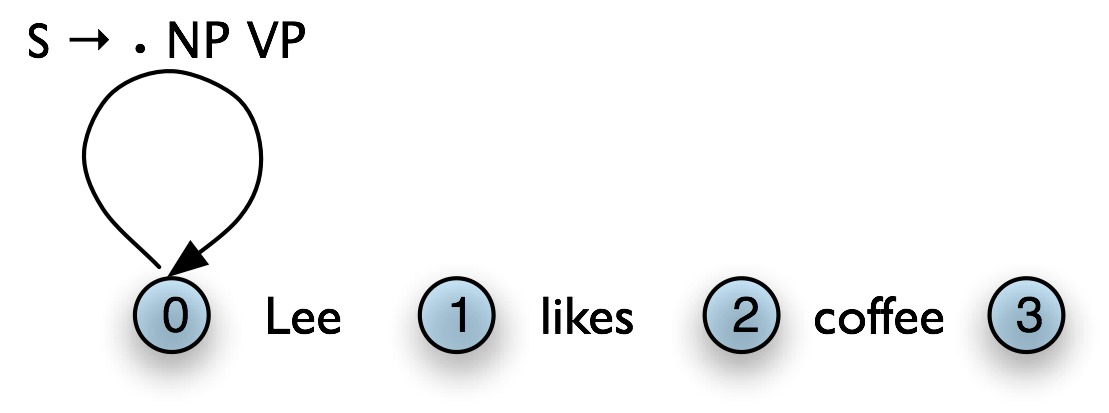As we mentioned before, the dot on the right hand side of a production records how far our goals have been satisfied. So in Figure 8.9(b), we are predicting that we will be able to find an np and a vp, but have not yet satisfied these subgoals. So how do we pursue them? In order to find an np, for instance, we need to invoke a production that has np on its left hand side. The step of adding the required edge to the chart is accomplished with the Top-Down Expand Rule (9). This tells us that if our chart contains an incomplete edge whose dot is followed by a nonterminal B, then the parser should add any self-loop edges licensed by the grammar whose left-hand side is B.

Thus, given a chart that looks like the one in Table 8.10(a), the Top-Down Expand Rule augments it with the edge shown in Table 8.10(b). In terms of our running example, we now have the chart shown in Table 8.10(c).

Table 8.10:

Top-Down Expand Rule

 a. Input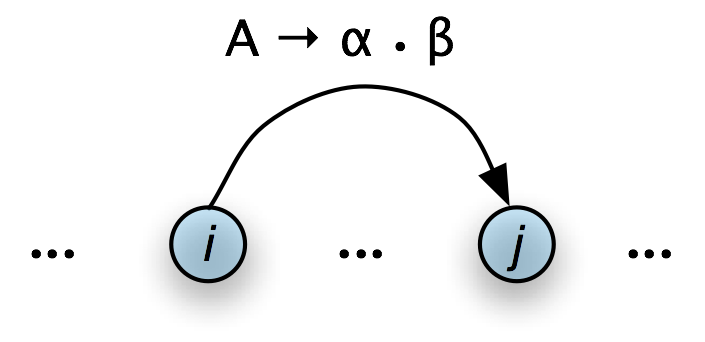b. Output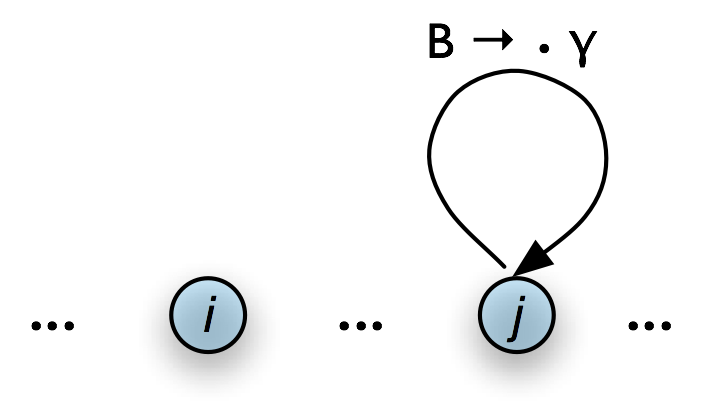c. Example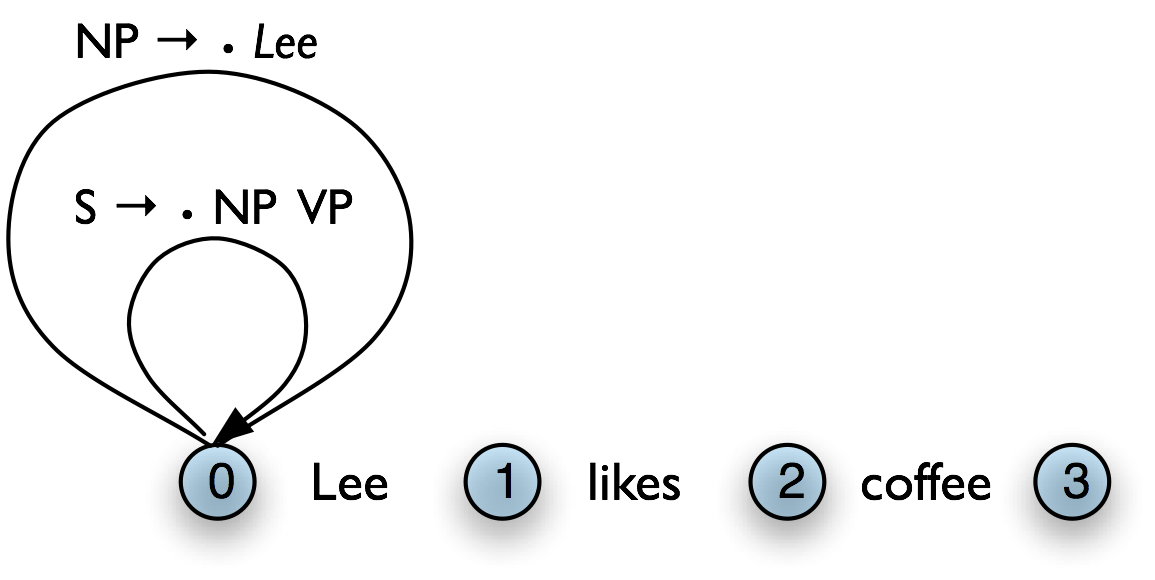The Top-Down Match rule allows the predictions of the grammar to be matched against the input string. Thus, if the chart contains an incomplete edge whose dot is followed by a terminal w, then the parser should add an edge if the terminal corresponds to the current input symbol.

Graphically, the Top-Down Match rule takes us from Table 8.11(a), to Table 8.11(b).

Table 8.11:

Top-Down Match Rule

 a. Inputb. OutputFigure 8.12(a) illustrates how our example chart after applying the Top-Down Match rule. What rule is relevant now? The Fundamental Rule. If we remove the self-loop edges from Figure 8.12(a) for simplicity, the Fundamental Rule gives us Figure 8.12(b).

Table 8.12:

Top-Down Example (cont)

 a. Apply Top-Down Match Ruleb. Apply Fundamental Rule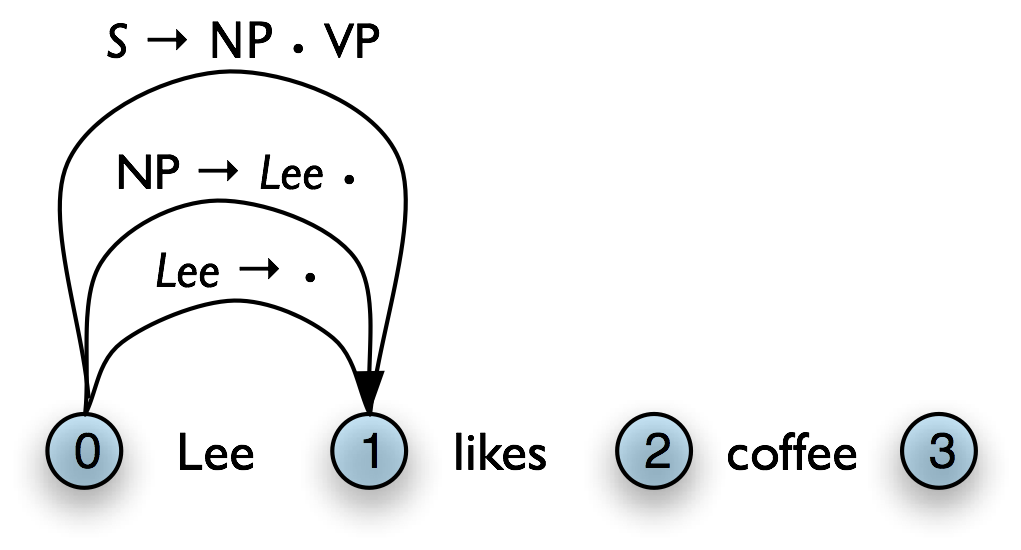Using these four rules, we can parse a sentence top-down as shown in (11).

We encourage you to experiment with the NLTK chart parser demo, as before, in order to test out the top-down strategy yourself.

### 8.3.5   The Earley Algorithm

The Earley algorithm [Earley, 1970] is a parsing strategy that resembles the Top-Down Strategy, but deals more efficiently with matching against the input string. Table 8.13 shows the correspondence between the parsing rules introduced above and the rules used by the Earley algorithm.

Table 8.13:

Terminology for rules in the Earley algorithm

Top-Down/Bottom-Up Earley
Top-Down Initialization Rule Top-Down Expand Rule Predictor Rule
Top-Down/Bottom-Up Match Rule Scanner Rule
Fundamental Rule Completer Rule

Let's look in more detail at the Scanner Rule. Suppose the chart contains an incomplete edge with a lexical category P immediately after the dot, the next word in the input is w, P is a part-of-speech label for w. Then the Scanner Rule admits a new complete edge in which P dominates w. More precisely:

To illustrate, suppose the input is of the form I saw ..., and the chart already contains the edge [vp → • v ..., (1, 1)]. Then the Scanner Rule will add to the chart the edges [v -> 'saw', (1, 2)] and ['saw'→ •, (1, 2)]. So in effect the Scanner Rule packages up a sequence of three rule applications: the Bottom-Up Initialization Rule for [w → •, (j, j+1)], the Top-Down Expand Rule for [P → • wj, (j, j)], and the Fundamental Rule for [Pwj •, (j, j+1))]. This is considerably more efficient than the Top-Down Strategy, that adds a new edge of the form [P → • w , (j, j)] for every lexical rule Pw, regardless of whether w can be found in the input. By contrast with Bottom-Up Initialization, however, the Earley algorithm proceeds strictly left-to-right through the input, applying all applicable rules at that point in the chart, and never backtracking. The NLTK chart parser demo, described above, allows the option of parsing according to the Earley algorithm.

### 8.3.6   Chart Parsing in NLTK

NLTK defines a simple yet flexible chart parser, ChartParser. A new chart parser is constructed from a grammar and a list of chart rules (also known as a strategy). These rules will be applied, in order, until no new edges are added to the chart. In particular, ChartParser uses the algorithm shown in (13).

 (13) ```Until no new edges are added: For each chart rule R: Apply R to any applicable edges in the chart. Return any complete parses in the chart. ```

nltk.parse.chart defines two ready-made strategies: TD_STRATEGY, a basic top-down strategy; and BU_STRATEGY, a basic bottom-up strategy. When constructing a chart parser, you can use either of these strategies, or create your own.

The following example illustrates the use of the chart parser. We start by defining a simple grammar, and tokenizing a sentence. We make sure it is a list (not an iterator), since we wish to use the same tokenized sentence several times.

 ```grammar = nltk.parse_cfg(''' NP -> NNS | JJ NNS | NP CC NP NNS -> "men" | "women" | "children" | NNS CC NNS JJ -> "old" | "young" CC -> "and" | "or" ''') parser = nltk.ChartParser(grammar, nltk.parse.BU_STRATEGY)```
 ```>>> sent = 'old men and women'.split() >>> for tree in parser.nbest_parse(sent): ... print tree (NP (JJ old) (NNS (NNS men) (CC and) (NNS women))) (NP (NP (JJ old) (NNS men)) (CC and) (NP (NNS women)))```

Listing 8.2 (chart_demo.py): Chart Parsing with NLTK

The trace parameter can be specified when creating a parser, to turn on tracing (higher trace levels produce more verbose output). Example 8.3 shows the trace output for parsing a sentence with the bottom-up strategy. Notice that in this output, '[-----]' indicates a complete edge, '>' indicates a self-loop edge, and '[----->' indicates an incomplete edge.

 ```>>> parser = nltk.ChartParser(grammar, nltk.parse.BU_STRATEGY, trace=2) >>> trees = parser.nbest_parse(sent) |. old . men . and . women .| Bottom Up Init Rule: |[---------] . . .| [0:1] 'old' |. [---------] . .| [1:2] 'men' |. . [---------] .| [2:3] 'and' |. . . [---------]| [3:4] 'women' Bottom Up Predict Rule: |> . . . .| [0:0] JJ -> * 'old' |. > . . .| [1:1] NNS -> * 'men' |. . > . .| [2:2] CC -> * 'and' |. . . > .| [3:3] NNS -> * 'women' Fundamental Rule: |[---------] . . .| [0:1] JJ -> 'old' * |. [---------] . .| [1:2] NNS -> 'men' * |. . [---------] .| [2:3] CC -> 'and' * |. . . [---------]| [3:4] NNS -> 'women' * Bottom Up Predict Rule: |> . . . .| [0:0] NP -> * JJ NNS |. > . . .| [1:1] NP -> * NNS |. > . . .| [1:1] NNS -> * NNS CC NNS |. . . > .| [3:3] NP -> * NNS |. . . > .| [3:3] NNS -> * NNS CC NNS Fundamental Rule: |[---------> . . .| [0:1] NP -> JJ * NNS |. [---------] . .| [1:2] NP -> NNS * |. [---------> . .| [1:2] NNS -> NNS * CC NNS |[-------------------] . .| [0:2] NP -> JJ NNS * |. [-------------------> .| [1:3] NNS -> NNS CC * NNS |. . . [---------]| [3:4] NP -> NNS * |. . . [--------->| [3:4] NNS -> NNS * CC NNS |. [-----------------------------]| [1:4] NNS -> NNS CC NNS * |. [-----------------------------]| [1:4] NP -> NNS * |. [----------------------------->| [1:4] NNS -> NNS * CC NNS |[=======================================]| [0:4] NP -> JJ NNS * Bottom Up Predict Rule: |. > . . .| [1:1] NP -> * NP CC NP |> . . . .| [0:0] NP -> * NP CC NP |. . . > .| [3:3] NP -> * NP CC NP Fundamental Rule: |. [---------> . .| [1:2] NP -> NP * CC NP |[-------------------> . .| [0:2] NP -> NP * CC NP |. . . [--------->| [3:4] NP -> NP * CC NP |. [----------------------------->| [1:4] NP -> NP * CC NP |[--------------------------------------->| [0:4] NP -> NP * CC NP |. [-------------------> .| [1:3] NP -> NP CC * NP |[-----------------------------> .| [0:3] NP -> NP CC * NP |. [-----------------------------]| [1:4] NP -> NP CC NP * |[=======================================]| [0:4] NP -> NP CC NP * |. [----------------------------->| [1:4] NP -> NP * CC NP |[--------------------------------------->| [0:4] NP -> NP * CC NP```

Listing 8.3 (butrace.py): Trace of Bottom-Up Parser

### 8.3.7   Exercises

1. ☼ Use the graphical chart-parser interface to experiment with different rule invocation strategies. Come up with your own strategy that you can execute manually using the graphical interface. Describe the steps, and report any efficiency improvements it has (e.g. in terms of the size of the resulting chart). Do these improvements depend on the structure of the grammar? What do you think of the prospects for significant performance boosts from cleverer rule invocation strategies?
2. ☼ We have seen that a chart parser adds but never removes edges from a chart. Why?
3. ◑ Write a program to compare the efficiency of a top-down chart parser compared with a recursive descent parser (Section 7.5.1). Use the same grammar and input sentences for both. Compare their performance using the timeit module (Section 5.5.4).

## 8.4   Probabilistic Parsing

As we pointed out in the introduction to this chapter, dealing with ambiguity is a key challenge to broad coverage parsers. We have shown how chart parsing can help improve the efficiency of computing multiple parses of the same sentences. But the sheer number of parses can be just overwhelming. We will show how probabilistic parsing helps to manage a large space of parses. However, before we deal with these parsing issues, we must first back up and introduce weighted grammars.

### 8.4.1   Weighted Grammars

We begin by considering the verb give. This verb requires both a direct object (the thing being given) and an indirect object (the recipient). These complements can be given in either order, as illustrated in example (14b). In the "prepositional dative" form, the indirect object appears last, and inside a prepositional phrase, while in the "double object" form, the indirect object comes first:

(14)

 a. Kim gave a bone to the dog

 b. Kim gave the dog a bone

Using the Penn Treebank sample, we can examine all instances of prepositional dative and double object constructions involving give, as shown in Listing 8.4.

 ```def give(t): return t.node == 'VP' and len(t) > 2 and t.node == 'NP'\ and (t.node == 'PP-DTV' or t.node == 'NP')\ and ('give' in t.leaves() or 'gave' in t.leaves()) def sent(t): return ' '.join(token for token in t.leaves() if token not in '*-0') def print_node(t, width): output = "%s %s: %s / %s: %s" %\ (sent(t), t.node, sent(t), t.node, sent(t)) if len(output) > width: output = output[:width] + "..." print output```
 ```>>> for tree in nltk.corpus.treebank.parsed_sents(): ... for t in tree.subtrees(give): ... print_node(t, 72) gave NP: the chefs / NP: a standing ovation give NP: advertisers / NP: discounts for maintaining or increasing ad sp... give NP: it / PP-DTV: to the politicians gave NP: them / NP: similar help give NP: them / NP: give NP: only French history questions / PP-DTV: to students in a Europe... give NP: federal judges / NP: a raise give NP: consumers / NP: the straight scoop on the U.S. waste crisis gave NP: Mitsui / NP: access to a high-tech medical product give NP: Mitsubishi / NP: a window on the U.S. glass industry give NP: much thought / PP-DTV: to the rates she was receiving , nor to ... give NP: your Foster Savings Institution / NP: the gift of hope and free... give NP: market operators / NP: the authority to suspend trading in futu... gave NP: quick approval / PP-DTV: to \$ 3.18 billion in supplemental appr... give NP: the Transportation Department / NP: up to 50 days to review any... give NP: the president / NP: such power give NP: me / NP: the heebie-jeebies give NP: holders / NP: the right , but not the obligation , to buy a cal... gave NP: Mr. Thomas / NP: only a `` qualified '' rating , rather than ``... give NP: the president / NP: line-item veto power```

Listing 8.4 (give.py): Usage of Give and Gave in the Penn Treebank sample

We can observe a strong tendency for the shortest complement to appear first. However, this does not account for a form like give NP: federal judges / NP: a raise, where animacy may be playing a role. In fact there turn out to be a large number of contributing factors, as surveyed by [Bresnan & Hay, 2006].

How can such tendencies be expressed in a conventional context free grammar? It turns out that they cannot. However, we can address the problem by adding weights, or probabilities, to the productions of a grammar.

 ```grammar = nltk.parse_pcfg(""" S -> NP VP [1.0] VP -> TV NP [0.4] VP -> IV [0.3] VP -> DatV NP NP [0.3] TV -> 'saw' [1.0] IV -> 'ate' [1.0] DatV -> 'gave' [1.0] NP -> 'telescopes' [0.8] NP -> 'Jack' [0.2] """)```
 ```>>> print grammar Grammar with 9 productions (start state = S) S -> NP VP [1.0] VP -> TV NP [0.4] VP -> IV [0.3] VP -> DatV NP NP [0.3] TV -> 'saw' [1.0] IV -> 'ate' [1.0] DatV -> 'gave' [1.0] NP -> 'telescopes' [0.8] NP -> 'Jack' [0.2]```

Listing 8.5 (pcfg1.py): Defining a Probabilistic Context Free Grammar (PCFG)

It is sometimes convenient to combine multiple productions into a single line, e.g. VP -> TV NP [0.4] | IV [0.3] | DatV NP NP [0.3]. In order to ensure that the trees generated by the grammar form a probability distribution, PCFG grammars impose the constraint that all productions with a given left-hand side must have probabilities that sum to one. The grammar in Listing 8.5 obeys this constraint: for S, there is only one production, with a probability of 1.0; for VP, 0.4+0.3+0.3=1.0; and for NP, 0.8+0.2=1.0. The parse tree returned by parse() includes probabilities:

 ```>>> viterbi_parser = nltk.ViterbiParser(grammar) >>> print viterbi_parser.parse(['Jack', 'saw', 'telescopes']) (S (NP Jack) (VP (TV saw) (NP telescopes))) (p=0.064)```

The next two sections introduce two probabilistic parsing algorithms for PCFGs. The first is an A* parser that uses Viterbi-style dynamic programming to find the single most likely parse for a given text. Whenever it finds multiple possible parses for a subtree, it discards all but the most likely parse. The second is a bottom-up chart parser that maintains a queue of edges, and adds them to the chart one at a time. The ordering of this queue is based on the probabilities associated with the edges, allowing the parser to expand more likely edges before less likely ones. Different queue orderings are used to implement a variety of different search strategies. These algorithms are implemented in the nltk.parse.viterbi and nltk.parse.pchart modules.